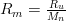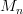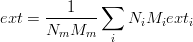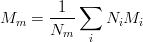# Thermochemistry database¶

This module implements all the necessary routines to access the Alexander Burcat’s Thermochemisty database. The database is included in the package.

To understand the module you should take a look at Third Millennium Ideal Gas and Condensed Phase Thermochemical Database for Combustion with Updates from Active Thermochemical Tables by Burcat A. and Ruscic B.

## thermopy.burcat¶

class thermopy.burcat.Element(formula, Tmin_, _Tmax, mm, hfr, elements)

Bases: object

This is a helper class. It is intended to be created via an Elementdb object but you can use it by your own. Take a look at Elementdb class.

Units are K, J, kg... conversion functions are provided in the external units module.

One extra feature not explained in Elementdb documentation is that it contains the number of each atom, useful for computing chemical reactions.

>>> db = Elementdb()
>>> weird = db.getelementdata("C8H6O2")
>>> print weird.elements
[('C', 8), ('H', 6), ('O', 2)]

cp
Computes the specific heat capacity in J/kg K at 298 K (Reference T)
cp_(T)
Computes the specific heat capacity in J/kg K for a given temperature
cpo(T)
Calculates the specific heat capacity in J/mol K
density(p, T)
Density.
go(T)
Computes the Gibbs free energy from the sensible enthalpy in J/mol
h(T)
Computes the total enthalpy in J/kg
ho(T)
Computes the sensible enthalpy in J/mol
so(T)
Computes enthropy in J/mol K
class thermopy.burcat.Elementdb

Bases: object

Class that reads the Alexander Burcat’s thermochemical database for combustion.

>>> db = Elementdb()
>>> oxygen = db.getelementdata("O2 REF ELEMENT")
>>> print oxygen
<element> O2 REF ELEMENT
>>> print 'molar mass',oxygen.mm
molar mass 0.0319988
>>> print 'heat capacity',oxygen.cp
heat capacity 918.078952423


The reference temperature for enthalpy is 298.15 K

>>> print 'enthalpy',oxygen.ho(298.15)
enthalpy 1.94293914332e-05
>>> print 'enthropy',oxygen.so(298)
enthropy 205.133745795
>>> print 'gibbs free energy',oxygen.go(298)
gibbs free energy -61134.2629008


There’s a search function. It is very useful because some names are a bit tricky. Well, not this one.

>>> db.search("AIR")
['AIR']
>>> air = db.getelementdata("AIR")
>>> print 'air molar mass',air.mm
air molar mass 0.02896518
>>> print 'heat capacity',air.cp
heat capacity 1004.77625096
>>> print air.density(101325,298)
1.1845186553


The element database can create also mixtures. It returns an instance of Mixture object that can give you the same as the Element class for any mixture.

>>> mix = db.getmixturedata([("O2 REF ELEMENT",20.9476),    ("N2  REF ELEMENT",78.084),    ("CO2",0.0319),    ("AR REF ELEMENT",0.9365),    ])
>>> print mix
<Mixture>:
O2 REF ELEMENT at 20.9476
N2  REF ELEMENT at 78.084
CO2 at 0.0319
AR REF ELEMENT at 0.9365
>>> print mix.cp
1004.72217065
>>> print mix.mm
0.028965116031

getelementdata(formula)
Returns an element instance given the name of the element.
getmixturedata(components)
Creates a mixture of components given a list of tuples containing the formula and the volume percent
search(formula)
List all the species containing a string. Helpful for interactive use of the database.
class thermopy.burcat.Mixture(config='vol')

Bases: object

Class that models a gas mixture. By now only volume (molar) composition is supported.

You can iterate through all its elements. The item returned is a tuple containing the element and the amount.

>>> db = Elementdb()
>>> mix = db.getmixturedata([("O2 REF ELEMENT",20.9476),    ("N2  REF ELEMENT",78.084),    ("CO2",0.0319),    ("AR REF ELEMENT",0.9365),    ])
>>> for e in mix: print e
(<element> O2 REF ELEMENT, 20.947600000000001)
(<element> N2  REF ELEMENT, 78.084000000000003)
(<element> CO2, 0.031899999999999998)
(<element> AR REF ELEMENT, 0.9365)


You can get elements either by index or by value.

>>> print mix['CO2']
(<element> CO2, 0.031899999999999998)


You can also delete components of a mixture. Needed by the MoistAir class

>>> mix.delete('CO2')
>>> print mix
<Mixture>:
O2 REF ELEMENT at 20.9476
N2  REF ELEMENT at 78.084
AR REF ELEMENT at 0.9365

cp
Computes the heat capacity
cp_(T)
Computes the heat capacity at a given temperature
delete(formula)
density(p, T)

Computes the density for a given mix of gases

The equivalent R for a mix is, whereis the equivalent molar mass for the mix.

extensive(attr, T)

Computes the extensive value for a mix. Remember that an extensive value depends on the amount of matter. Enthalpy and volume are extensive values.go(T)
h(T)
ho(T)
mm

Computes the equivalent molar mass for a mixnext()
so(T)